# Object Tracking and Motion Planning Using Frenet Reference Path

This example shows you how to dynamically replan the motion of an autonomous vehicle based on the estimate of the surrounding environment. You use a Frenet reference path and a joint probabilistic data association (JPDA) tracker to estimate and predict the motion of other vehicles on the highway. Compared to the Highway Trajectory Planning Using Frenet Reference Path example, you use these estimated trajectories from the multi-object tracker in this example instead of ground truth for motion planning.

### Introduction

Dynamic replanning for autonomous vehicles is typically done with a local motion planner. The local motion planner is responsible for generating optimal trajectory based on a global plan and real-time information about the surrounding environment. The global plan for highway trajectory planning can be described as a pre-generated coordinate list of the highway centerline. The surrounding environment can be described mainly in two ways:

1. Discrete set of objects in the surrounding environment with defined geometries.

2. Discretized grid with estimates about free and occupied regions in the surrounding environment.

In the presence of dynamic obstacles, a local motion planner also requires predictions about the surroundings to assess the validity of planned trajectories. In this example, you represent the surrounding environment using the discrete set of objects approach. For an example using discretized grid, refer to the Motion Planning in Urban Environments Using Dynamic Occupancy Grid Map (Sensor Fusion and Tracking Toolbox) example.

#### Object State Transition and Measurement Modeling

The object list and their future predictions for motion planning are typically estimated by a multi-object tracker. The multi-object tracker accepts data from sensors and estimates the list of objects. In the tracking community, this list of objects is often termed as track list.

In this example, you use radar and camera sensors and estimate the track list using a JPDA multi-object tracker. The first step towards using any multi-object tracker is defining the object state, how the state evolves with time (state transition model) and how the sensor perceives it (measurement model). Common state transition models include constant-velocity model, constant-acceleration model etc. However, in the presence of map information, road network can be integrated into the motion model. In this example, you use a Frenet coordinate system to describe the object state at any given time step, $\mathit{k}$.

`${\mathit{x}}_{\mathit{k}}=\left[{\mathit{s}}_{\mathit{k}}\stackrel{˙}{\text{\hspace{0.17em}}{\mathit{s}}_{\mathit{k}\text{\hspace{0.17em}}}\text{\hspace{0.17em}}}{\mathit{d}}_{\mathit{k}\text{\hspace{0.17em}}}\text{\hspace{0.17em}}\stackrel{˙}{{\mathit{d}}_{\mathit{k}\text{\hspace{0.17em}}}}\right]$`

where ${\mathit{s}}_{\mathit{k}}$ and ${\mathit{d}}_{\mathit{k}\text{\hspace{0.17em}}}$represents the distance of the object along and perpendicular to highway centerline, respectively. You use a constant-speed state transition model to describe the object motion along the highway and a decaying-speed model to describe the motion perpendicular to the highway centerline. This decaying speed model allows you to represent lane change maneuvers by other vehicles on the highway.

`$\left[\begin{array}{c}{\mathit{s}}_{\mathit{k}+1}\\ \stackrel{˙}{{\mathit{s}}_{\mathit{k}+1}}\\ {\mathit{d}}_{\mathit{k}+1}\\ \stackrel{˙}{{\mathit{d}}_{\mathit{k}+1}}\end{array}\right]=\left[\begin{array}{cccc}1& \Delta \mathit{T}& 0& 0\\ 0& 1& 0& 0\\ 0& 0& 1& \tau \left(1-{\mathit{e}}^{\left(-\frac{\Delta \mathit{T}}{\text{\hspace{0.17em}}\tau }\right)\text{\hspace{0.17em}}}\right)\\ 0\text{\hspace{0.17em}}& 0\text{\hspace{0.17em}}& 0\text{\hspace{0.17em}}& {\mathit{e}}^{\left(-\frac{\Delta \mathit{T}}{\text{\hspace{0.17em}}\tau }\right)\text{\hspace{0.17em}}}\end{array}\right]\left[\begin{array}{c}{\mathit{s}}_{\mathit{k}}\\ \stackrel{˙}{{\mathit{s}}_{\mathit{k}}}\\ {\mathit{d}}_{\mathit{k}}\\ \stackrel{˙}{{\mathit{d}}_{\mathit{k}}}\end{array}\right]+\left[\begin{array}{cc}\frac{\Delta {\mathit{T}}^{2}}{2}& 0\\ \Delta \mathit{T}& 0\\ 0& \frac{\Delta {\mathit{T}}^{2}}{2}\\ 0& \Delta \mathit{T}\end{array}\right]\left[\begin{array}{c}{\mathit{w}}_{\mathit{s}}\\ {\mathit{w}}_{\mathit{d}\text{\hspace{0.17em}}}\end{array}\right]$`

where $\Delta \mathit{T}$ is the time difference between steps $\mathit{k}$ and $\mathit{k}+1$, ${\mathit{w}}_{\mathit{s}}$ and ${\mathit{w}}_{\mathit{d}\text{\hspace{0.17em}}}$ are zero-mean Gaussian noise representing unknown acceleration in Frenet coordinates, and $\tau$ is a decaying constant.

This choice of coordinate in modeling the object motion allows you to integrate the highway reference path into the multi-object tracking framework. The integration of reference path acts as additional information for the tracker and allows the tracker to improve current state estimates as well as predicted trajectories of the estimated objects. You can obtain measurement model by first transforming the object state into Cartesian position and velocity and then converting them to respective measured quantities such as azimuth and range.

### Setup

#### Scenario and Sensors

The scenario used in this example is created using the Driving Scenario Designer (Automated Driving Toolbox) and then exported to a MATLAB® function. The ego vehicle is mounted with 1 forward-looking radar and 5 cameras providing 360-degree coverage. The radar and cameras are simulated using the `drivingRadarDataGenerator` (Automated Driving Toolbox) and `visionDetectionGenerator` (Automated Driving Toolbox) System objects, respectively.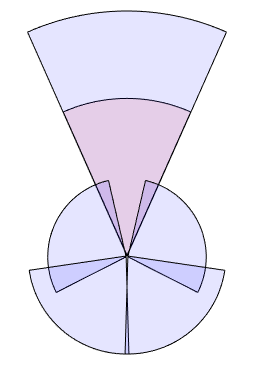The entire scenario and sensor setup is defined in the helper function, `helperTrackingAndPlanningScenario`, attached with this example. You define the global plan describing the highway centerline using a `referencePathFrenet` object. As multiple algorithms in this example need access to the reference path, you define the `helperGetReferencePath` function, which uses a `persistent` object that can be accessed by any function.

```rng(2022); % For reproducible results % Setup scenario and sensors [scenario, egoVehicle, sensors] = helperTrackingAndPlanningScenario();```

#### Joint Probabilistic Data Association Tracker

You set up a joint probabilistic data association tracker using the `trackerJPDA` (Sensor Fusion and Tracking Toolbox) System object. You set the `FilterInitializationFcn` property of the tracker to `helperInitRefPathFilter` function. This helper function defines an extended Kalman filter, `trackerJPDA` (Sensor Fusion and Tracking Toolbox), used to estimate the state of a single object. Local functions inside the `helperInitRefPathFilter `file define the state transition as well as measurement model for the filter. Further, to predict the tracks at a future time for the motion planner, you use the `predictTracksToTime` (Sensor Fusion and Tracking Toolbox) function of the tracker.

```tracker = trackerJPDA('FilterInitializationFcn',@helperInitRefPathFilter,... 'AssignmentThreshold',[200 inf],... 'ConfirmationThreshold',[8 10],... 'DeletionThreshold',[5 5]);```

#### Motion Planner

You use a similar highway trajectory motion planner as outlined in the Highway Trajectory Planning Using Frenet Reference Path example. The motion planner uses a planning horizon of 5 seconds and considers three modes for sampling trajectories for the ego vehicle — cruise control, lead vehicle follow, and basic lane change. The entire process for generating an optimal trajectory is wrapped in the helper function, `helperPlanHighwayTrajectory`.

The helper function accepts an `dynamicCapsuleList` object as an input to find non-colliding trajectories. The collision checking is performed in the entire planning horizon at an interval of 0.5 seconds. As the track states vary with time, you update the `dynamicCapsuleList` object in the simulation loop using the `helperUpdateCapsuleList` function, attached with this example.

```% Collision check time stamps tHorizon = 5; % seconds deltaT = 0.5; % seconds tSteps = deltaT:deltaT:tHorizon; % Create the dynamicCapsuleList object capList = dynamicCapsuleList; capList.MaxNumSteps = numel(tSteps) + 1; % Specify the ego vehicle geometry carLen = 4.7; carWidth = 1.8; rearAxleRatio = 0.25; egoID = 1; [egoID, egoGeom] = egoGeometry(capList,egoID); % Inflate to allow uncertainty and safety gaps egoGeom.Geometry.Length = 2*carLen; % in meters egoGeom.Geometry.Radius = carWidth/2; % in meters egoGeom.Geometry.FixedTransform(1,end) = -2*carLen*rearAxleRatio; % in meters updateEgoGeometry(capList,egoID,egoGeom);```

### Run Simulation

In this section, you advance the simulation, generate sensor data and perform dynamic replanning using estimations about the surroundings. The entire process is divided into 5 main steps:

1. You collect simulated sensor data from radar and camera sensors.

2. You feed the sensor data to the JPDA tracker to estimate current state of objects.

3. You predict the state of objects using the `predictTracksToTime` function.

4. You update the object list for the planner and plan a highway trajectory.

5. You move the simulated ego vehicle on the planned trajectory.

```% Create display for visualizing results display = HelperTrackingAndPlanningDisplay; % Initial state of the ego vehicle refPath = helperGetReferencePath; egoState = frenet2global(refPath,[0 0 0 0.5*3.6 0 0]); helperMoveEgoToState(egoVehicle, egoState); while advance(scenario) % Current time time = scenario.SimulationTime; % Step 1. Collect data detections = helperGenerateDetections(sensors, egoVehicle, time); % Step 2. Feed detections to tracker tracks = tracker(detections, time); % Step 3. Predict tracks in planning horizon timesteps = time + tSteps; predictedTracks = repmat(tracks,[1 numel(timesteps)+1]); for i = 1:numel(timesteps) predictedTracks(:,i+1) = predictTracksToTime(tracker,'confirmed',timesteps(i)); end % Step 4. Update capsule list and plan highway trajectory currActorState = helperUpdateCapsuleList(capList, predictedTracks); [optimalTrajectory, trajectoryList] = helperPlanHighwayTrajectory(capList, currActorState, egoState); % Visualize the results display(scenario, egoVehicle, sensors, detections, tracks, capList, trajectoryList); % Step 5. Move ego on planned trajectory egoState = optimalTrajectory(2,:); helperMoveEgoToState(egoVehicle,egoState); end```

### Results

In the animation below, you can observe the planned ego vehicle trajectories highlighted in green color. The animation also shows all other sampled trajectories for the ego vehicle. For these other trajectories, the colliding trajectories are shown in red, unevaluated trajectories are shown in grey, and kinematically-infeasible trajectories are shown in cyan color. Each track is annotated by an ID representing its unique identity. Notice that the ego vehicle successfully maneuvers around obstacles in the scene.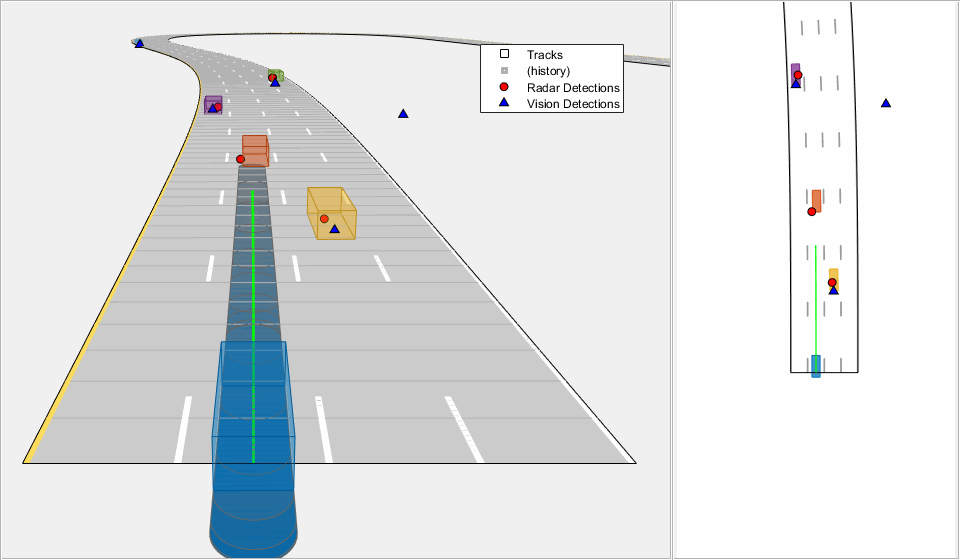In the following sub-sections, you analyze the estimates from the tracker at certain time steps and understand how it impacts the choices made by the motion planner.

In this section, you learn how the road-integrated motion model allows the tracker to obtain more accurate long-term predictions about the objects on the highway. Shown below is a snapshot from the simulation taken at time = 30 seconds. Notice the trajectory predicted for the green vehicle to the right of the blue ego vehicle. The predicted trajectory follows the lane of the vehicle because the road network information is integrated with the tracker. If instead, you use a constant-velocity model assumption for objects, the predicted trajectory will follow the direction of instantaneous velocity and will be falsely treated as a collision by the motion planner. In this case, the motion planner can possibly generate an unsafe maneuver.

`showSnaps(display,2,4); % Shows snapshot while publishing`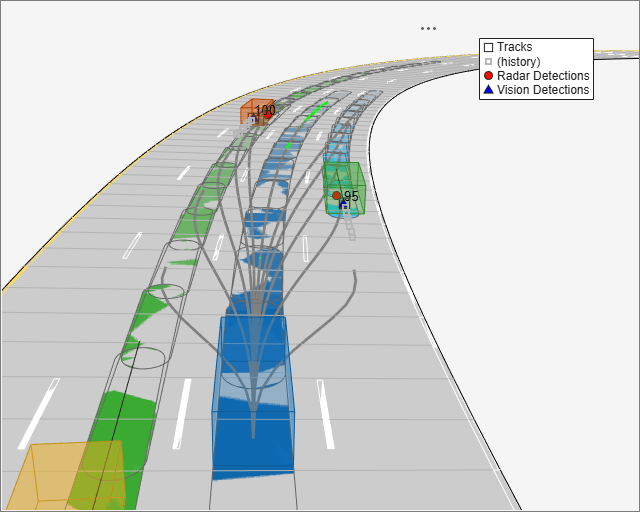#### Lane change prediction

In the first section, you learned how the lane change maneuvers are captured by using a decaying lateral velocity model of the objects. Now, notice the snapshot taken at time = 17.5 seconds. At this time, the yellow vehicle on the right side of the ego vehicle initiates a lane change and intends to enter the lane of the ego vehicle. Notice that its predicted trajectory captures this maneuver, and the tracker predicts it to be in the same lane as the ego vehicle at the end of planning horizon. This prediction informs the motion planner about a possible collision with this vehicle, thus the planner first proceeds to test feasibility for the ego vehicle to change lane to the left. However, the presence of purple vehicle on the left and its predicted trajectory causes the ego vehicle to make a right lane change. You can also observe these colliding trajectories colored as red in the snapshot below.

`showSnaps(display,2,1); % Shows snapshot while publishing`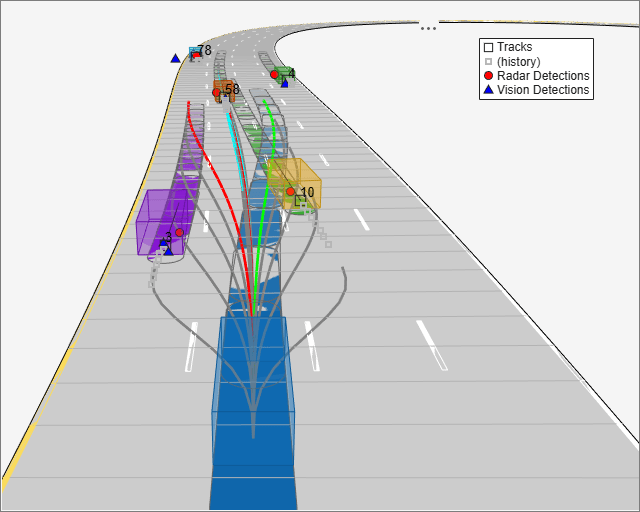#### Tracker imperfections

A multi-object tracker may have certain imperfections that can affect motion planning decisions. Specifically, a multi-object tracker can miss objects, report false tracks, or sometimes report redundant tracks. In the snapshot below taken at time = 20 seconds, the tracker drops tracks on two vehicles in front of the ego vehicle due to occlusion. In this particular situation, these missed targets are less likely to influence the decision of the motion planner due to their distance from the ego vehicle.

`showSnaps(display,2,2); % Shows snapshot while publishing`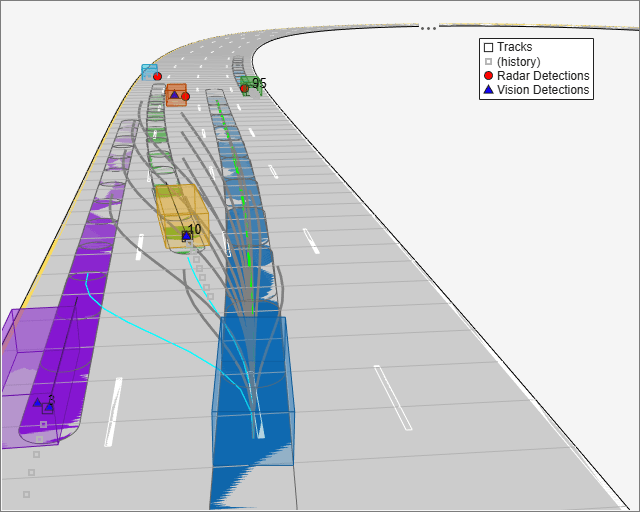However, as the ego vehicle approaches these vehicles, their influence on the ego vehicle's decision increases. Notice that the tracker is able to establish a track on these vehicles by time = 20.4 seconds, as shown in the snapshot below, thus making the system slightly robust to these imperfections. While configuring a tracking algorithm for motion planning, it is important to consider these imperfections from the tracker and tune the track confirmation and track deletion logics.

`showSnaps(display,2,3); % Show snapshot while publishing`### Summary

You learned how to use a joint probabilistic data association tracker to track vehicles using a Frenet reference path with radar and camera sensors. You configured the tracker to use highway map data to provide long term predictions about objects. You also used these long-term predictions to drive a motion planner for planning trajectories on the highway.

### Supporting Functions

```function detections = helperGenerateDetections(sensors, egoVehicle, time) detections = cell(0,1); for i = 1:numel(sensors) thisDetections = sensors{i}(targetPoses(egoVehicle),time); detections = [detections;thisDetections]; %#ok<AGROW> end detections = helperAddEgoVehicleLocalization(detections,egoVehicle); detections = helperPreprocessDetections(detections); end function detectionsOut = helperAddEgoVehicleLocalization(detectionsIn, egoPose) defaultParams = struct('Frame','Rectangular',... 'OriginPosition',zeros(3,1),... 'OriginVelocity',zeros(3,1),... 'Orientation',eye(3),... 'HasAzimuth',false,... 'HasElevation',false,... 'HasRange',false,... 'HasVelocity',false); fNames = fieldnames(defaultParams); detectionsOut = cell(numel(detectionsIn),1); for i = 1:numel(detectionsIn) thisDet = detectionsIn{i}; if iscell(thisDet.MeasurementParameters) measParams = thisDet.MeasurementParameters{1}; else measParams = thisDet.MeasurementParameters(1); end newParams = struct; for k = 1:numel(fNames) if isfield(measParams,fNames{k}) newParams.(fNames{k}) = measParams.(fNames{k}); else newParams.(fNames{k}) = defaultParams.(fNames{k}); end end % Add parameters for ego vehicle thisDet.MeasurementParameters = [newParams;newParams]; thisDet.MeasurementParameters(2).Frame = 'Rectangular'; thisDet.MeasurementParameters(2).OriginPosition = egoPose.Position(:); thisDet.MeasurementParameters(2).OriginVelocity = egoPose.Velocity(:); thisDet.MeasurementParameters(2).Orientation = rotmat(quaternion([egoPose.Yaw egoPose.Pitch egoPose.Roll],'eulerd','ZYX','frame'),'frame')'; % No information from object class and attributes thisDet.ObjectClassID = 0; thisDet.ObjectAttributes = struct; detectionsOut{i} = thisDet; end end function detections = helperPreprocessDetections(detections) % This function pre-process the detections from radars and cameras to % fit the modeling assumptions used by the tracker % 1. It removes velocity information from camera detections. This is % because those are filtered estimates and the assumptions from camera % may not align with defined prior information for tracker. % % 2. It fixes the bias for camera sensors that arise due to camera % projections for cars just left or right to the ego vehicle. % % 3. It inflates the measurement noise for range-rate reported by the % radars to match the range-rate resolution of the sensor for i = 1:numel(detections) if detections{i}.SensorIndex > 1 % Camera % Remove velocity detections{i}.Measurement = detections{i}.Measurement(1:3); detections{i}.MeasurementNoise = blkdiag(detections{i}.MeasurementNoise(1:2,1:2),25); detections{i}.MeasurementParameters(1).HasVelocity = false; % Fix bias pos = detections{i}.Measurement(1:2); if abs(pos(1)) < 5 && abs(pos(2)) < 5 [az, ~, r] = cart2sph(pos(1),pos(2),0); [pos(1),pos(2)] = sph2cart(az, 0, r + 0.7); % Increase range detections{i}.Measurement(1:2) = pos; detections{i}.MeasurementNoise(2,2) = 0.25; end else % Radars detections{i}.MeasurementNoise(3,3) = 0.5^2/4; end end end ```
```function helperMoveEgoToState(egoVehicle, egoState) egoVehicle.Position(1:2) = egoState(1:2); egoVehicle.Velocity(1:2) = [cos(egoState(3)) sin(egoState(3))]*egoState(5); egoVehicle.Yaw = egoState(3)*180/pi; egoVehicle.AngularVelocity(3) = 180/pi*egoState(4)*egoState(5); end```